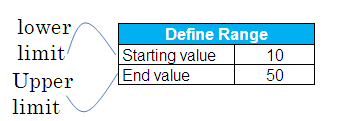# How to count prime numbers with Excel

Scenario

Suppose you are asked to find out the number of prime numbers in an interval in MS Excel. A prime number is a number that cannot be evenly divided by any other number except 1 and itself. For example, some prime numbers are 2, 3, 5, 7, 11, 13, 17, and so on. These numbers have no divisors other than 1 and the number itself.Suppose lower limit of a range is entered in cell C2 and upper limit in cell C3.
Solution
1. Press CTRL + F3 to open the Name Manager dialog box.
2. Click on the "New" button.
3. In the "Name" field, type rng.
4. In the "Refers to:" box, enter =ROW(INDIRECT(Sheet1!\$C\$2&":"&Sheet1!\$C\$3))
5. Click OK
6. Click CloseIn cell E5, enter the following formula :

`=SUM(IF(MMULT(--(IF(rng>TRANSPOSE(rng-C2+2),MOD(rng,(rng>TRANSPOSE(rng-C2+2))*TRANSPOSE(rng-C2+2)))=0),rng-C2+2)=0,1))`

Hit CTRL + SHIFT + ENTER to confirm this formula as an array formula. If done correctly, Excel will automatically place curly braces {...} around the formula.

Related Post :
Generating prime numbers in an interval with Excel
Check if a number is prime with Excel
Related Posts1.